# Perform Left/Mid On Array Formula (Excel)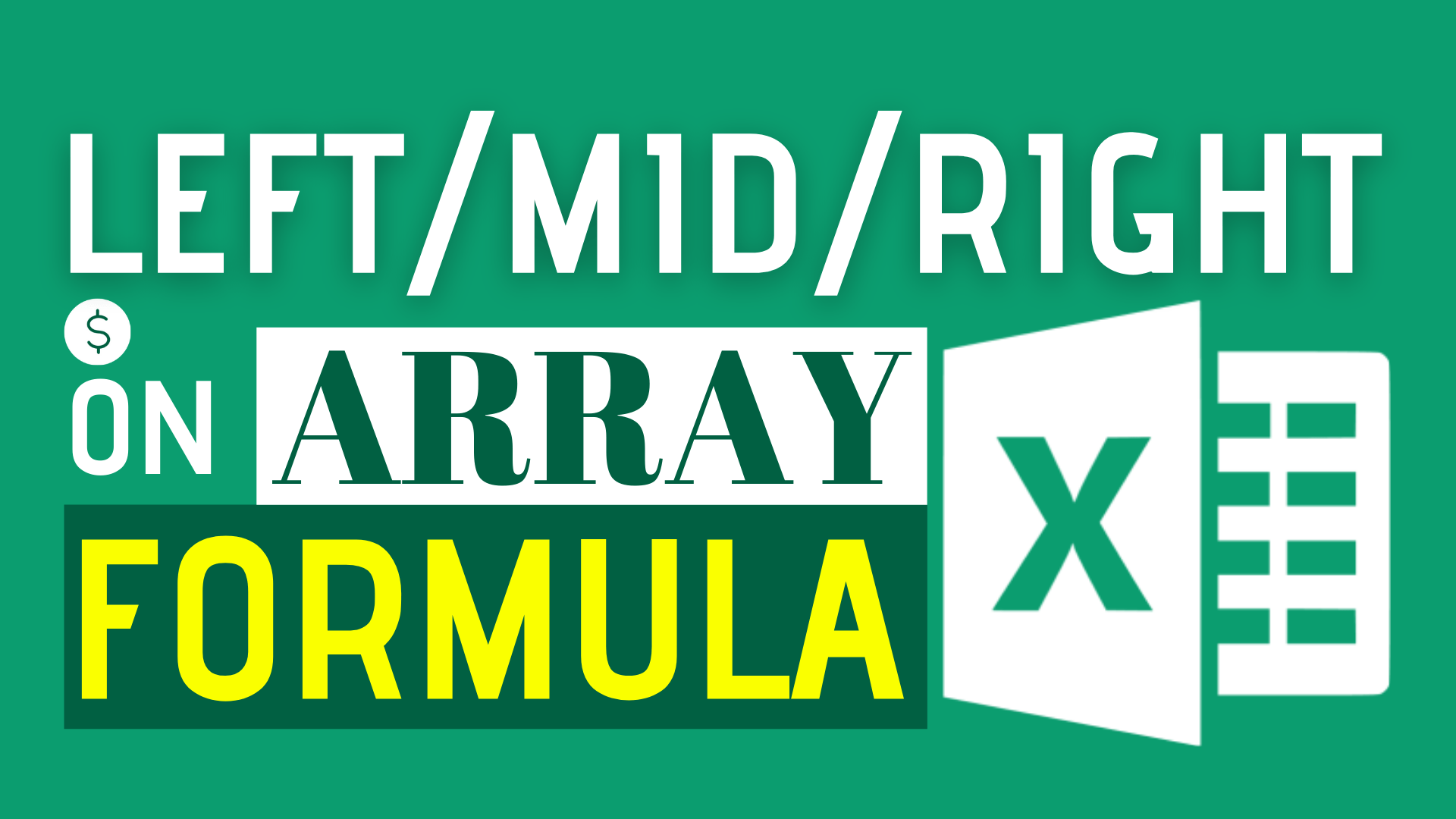LEFT, MID, RIGHT are some of the most useful text function in Excel. They allow us to extract a specified number of characters from a text string.

Yet, normal LEFT, MID, RIGHT only work in a single cell.

To perform those functions on Array formula, you will need to perform a trick.

Do you have the following questions?

1. How to perform Text function with array formula?
2. How to use Left or Right function along with array formula?
3. Can I use array formula with left right mid function?

In this article, I will show you how to perform LEFT/MID with Array Formula in Excel.

## Example

In this example, there are two tables.

One is information table. One is Department lookup table.

Employee ID consists of two parts. The text indicates the department where the employee belongs to.

What do we want to find out?

We want to find out the department each employee belong to.

## Formula of Cell C3

`=INDEX('Sample data'!\$F\$3:\$F\$10,MATCH(LEFT(B3,3),RIGHT(\$E\$3:\$E\$10,3),0))`

This is an array formula. Instead of enter, remember to press CTRL + SHIFT + ENTER while using it.

Explanation

To get the department information, we will need to extract the leftmost 3 characters from [Employee ID] and the rightmost 3 characters from [Code] to do a matching.

In order to do that, we need to use the LEFT and RIGHT function.

Performing LEFT function on [Employee ID] column is no different from the usual way we perform.

But performing RIGHT function on [Code] column is a bit tricky since you are trying to perform the text function on an array formula.

Instead of matching a cell with a cell, doing INDEX MATCH means you are matching a cell with an array.

In this situation, you need to press CTRL + SHIFT + ENTER.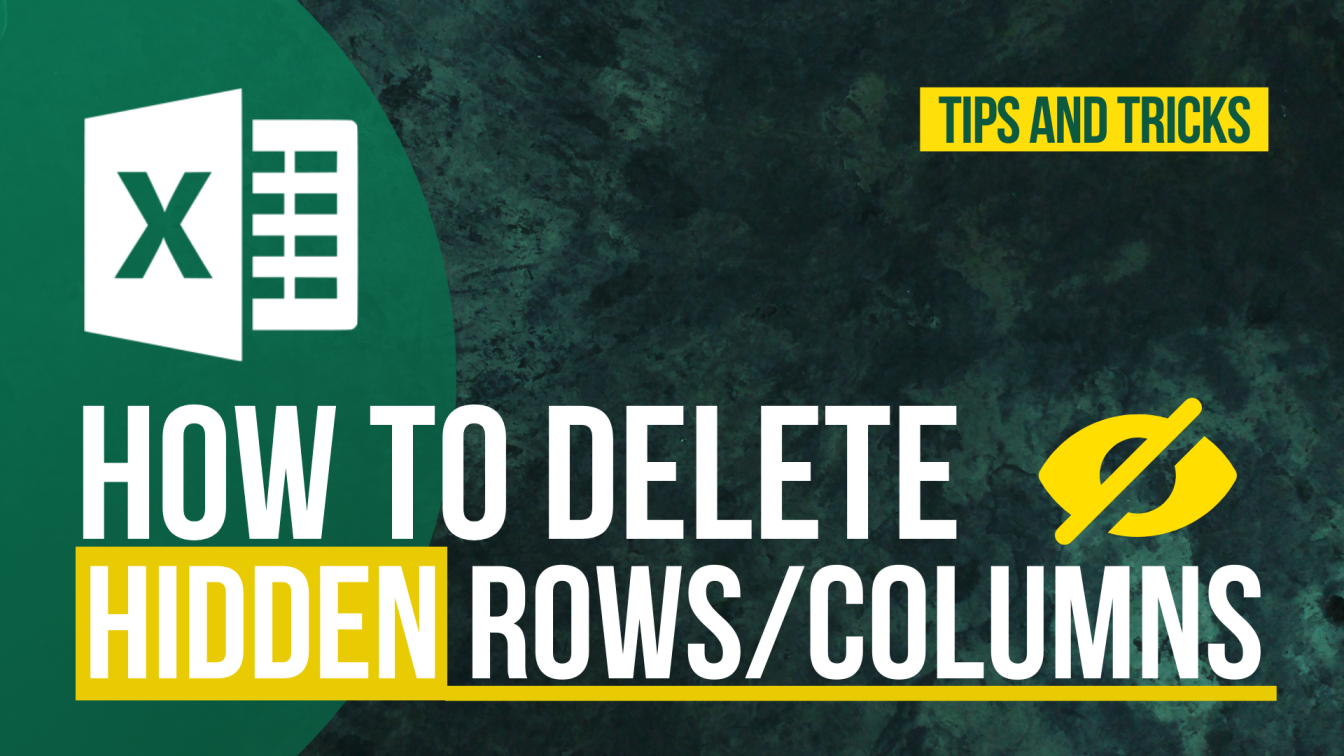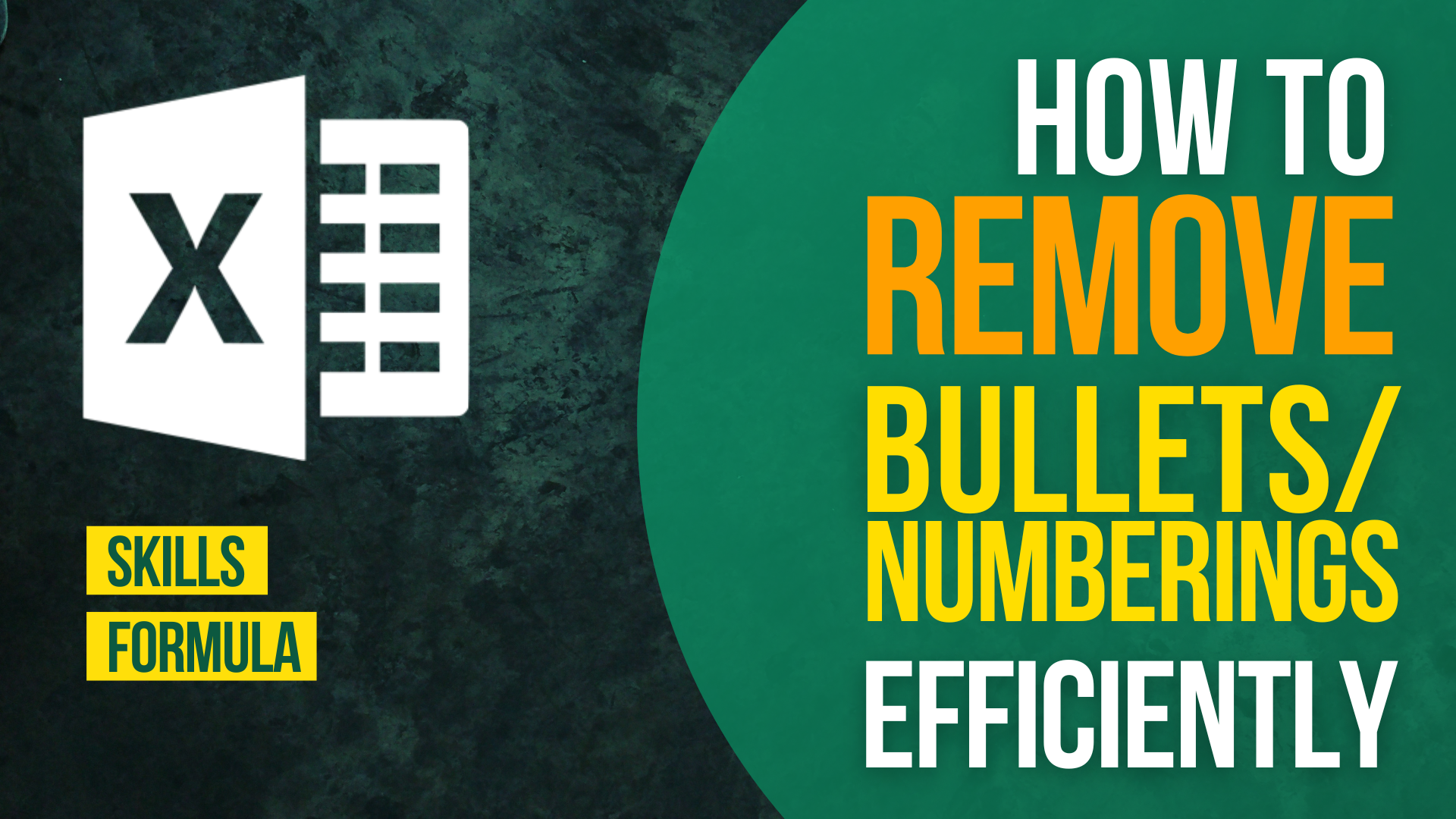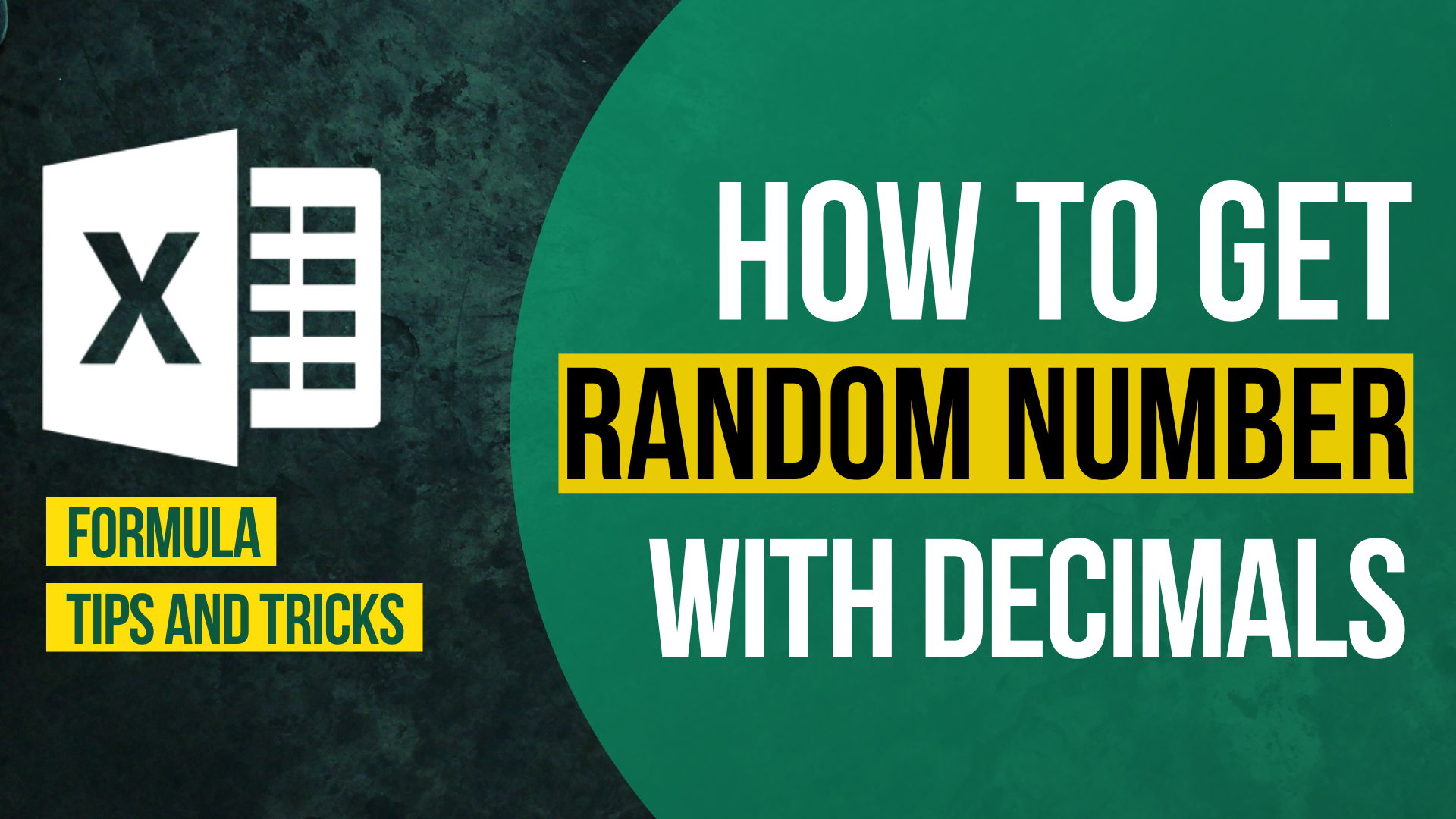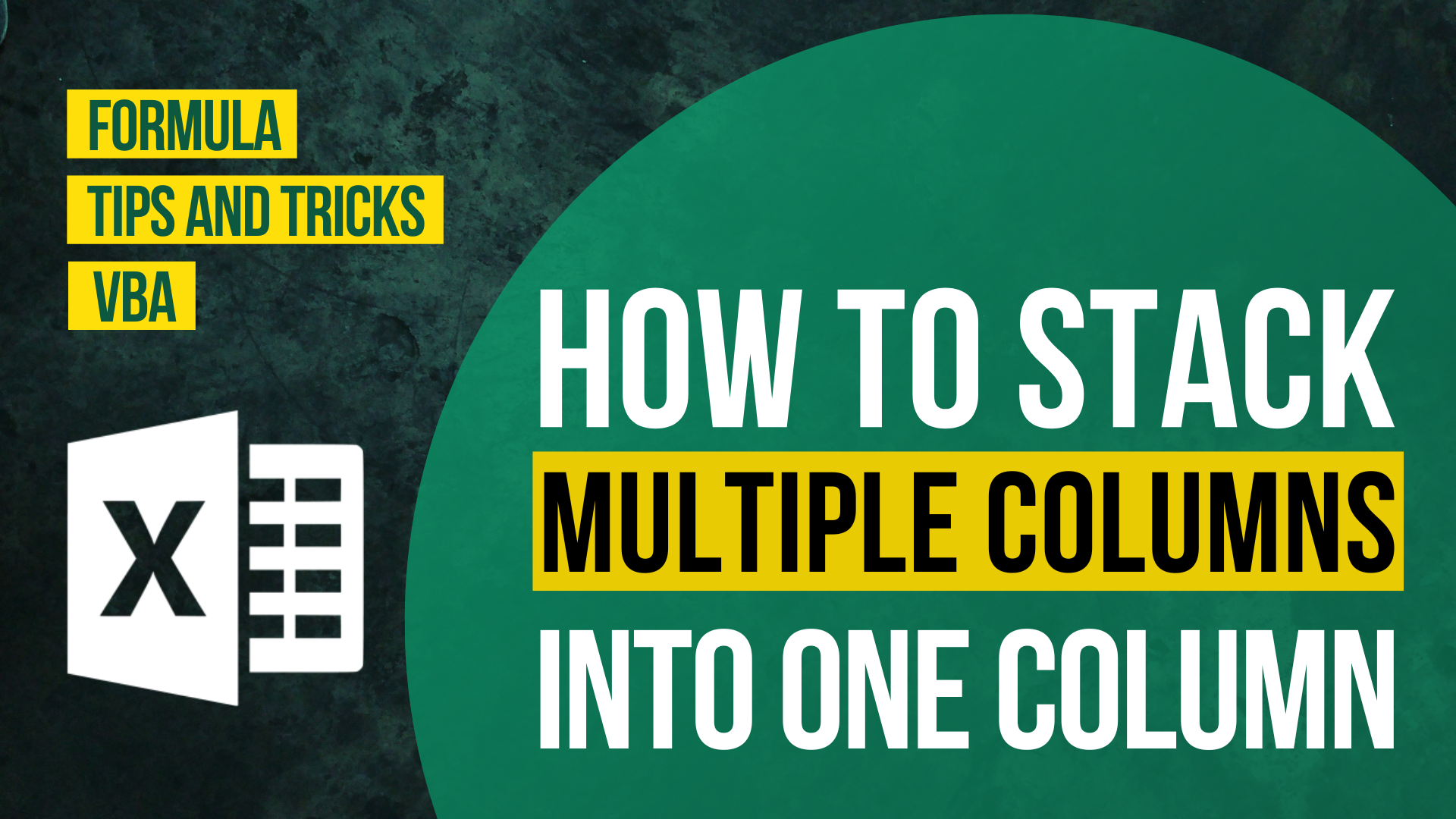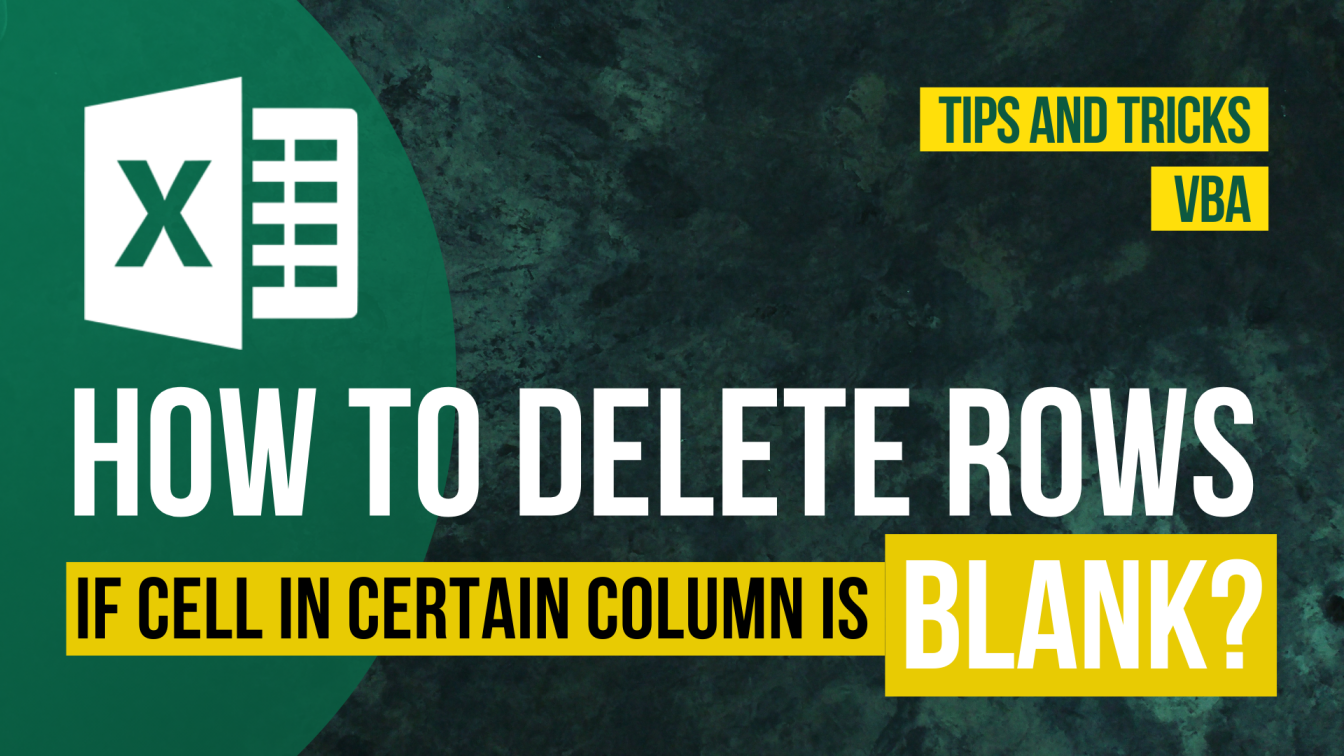How to Input Time Duration in Excel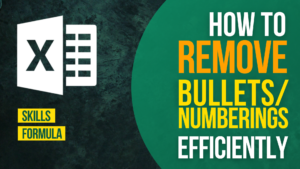How to Remove Bullets/Numberings in Excel

Popular posts